#《Scikit-Learn与TensorFlow机器学习实用指南》第14章 循环神经网络

0
0
01. 云栖社区>
2. 博客>
3. 正文

## 《Scikit-Learn与TensorFlow机器学习实用指南》第14章 循环神经网络

apachecn_飞龙 2018-05-17 20:58:05 浏览1807

# 第14章 循环神经网络

## 循环神经元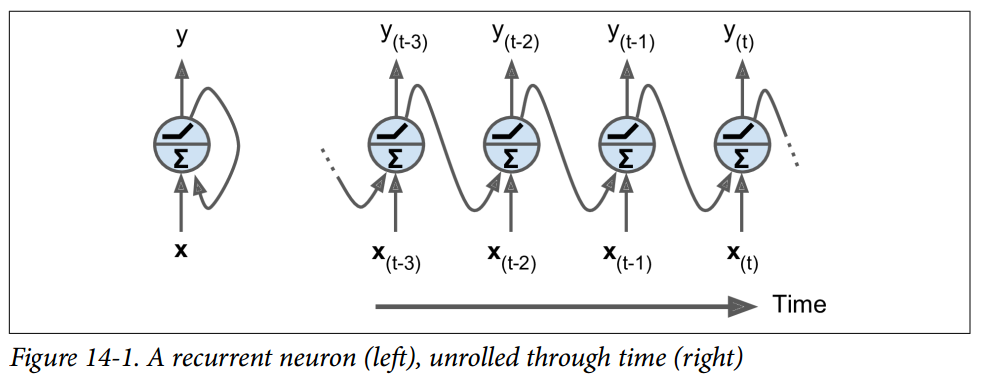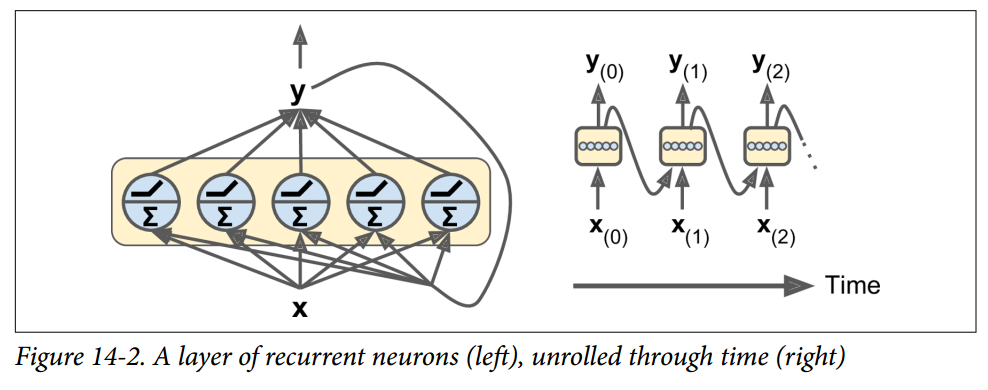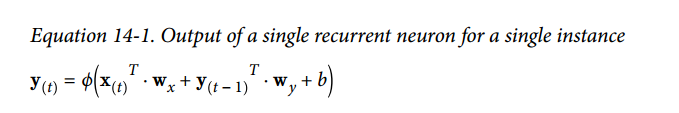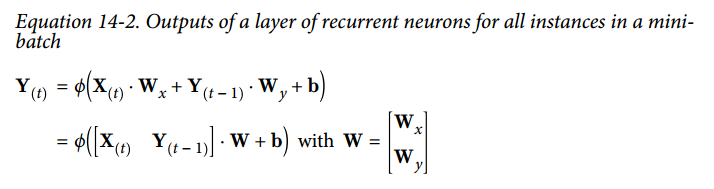• Y(t)Y(t)是m \times n_{neurons}tm矩阵，包含在最小批次中每个实例在时间步‘t‘处的层输出（‘m‘是小批次中的实例数，n_{neurons}\$ 是神经元数）。
• X(t)X(t)m×ninputsm×ninputs 矩阵，包含所有实例的输入的 （ninputsninputs 是输入特征的数量）。
• WxWxninputs×nneuronsninputs×nneurons 矩阵，包含当前时间步的输入的连接权重的。
• WyWynneurons×nneuronsnneurons×nneurons 矩阵，包含上一个时间步的输出的连接权重。
• 权重矩阵 WxWxWyWy 通常连接成单个权重矩阵W，形状为 (ninputs+nneurons)×nneurons(ninputs+nneurons)×nneurons（见公式 14-2 的第二行）
• b是大小为 nneuronsnneurons 的向量，包含每个神经元的偏置项。

## 记忆单元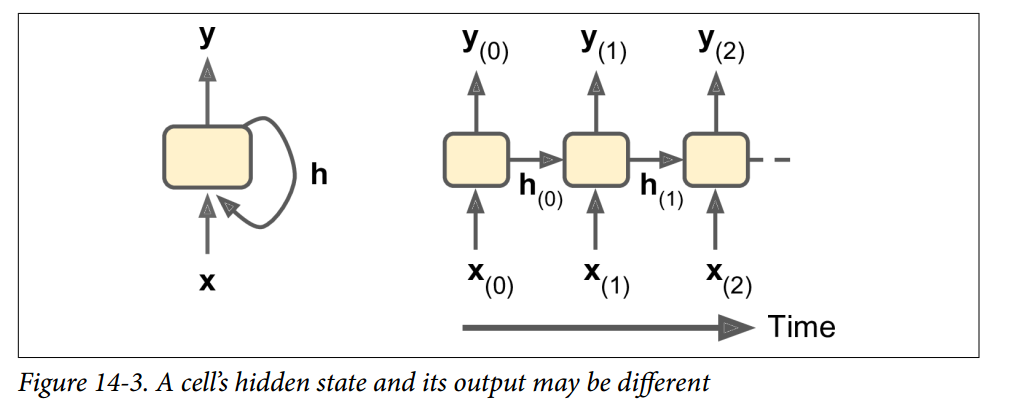## 输入和输出序列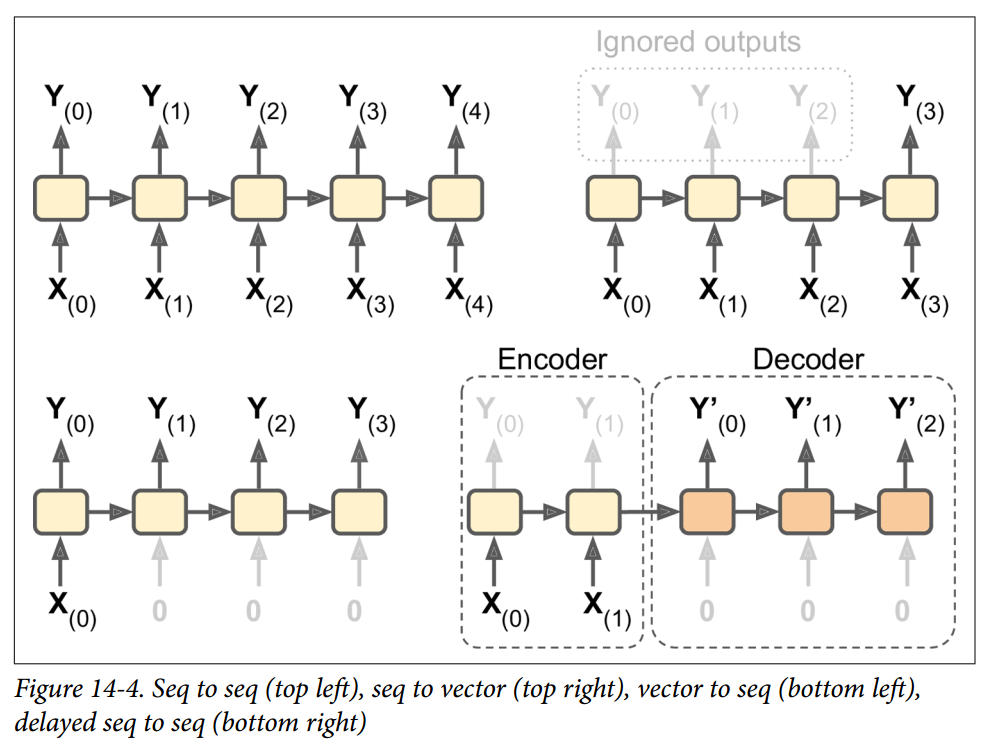RNN 可以同时进行一系列输入并产生一系列输出（见图 14-4，左上角的网络）。 例如，这种类型的网络对于预测时间序列（如股票价格）非常有用：你在过去的N天内给出价格，并且它必须输出向未来一天移动的价格（即从N - 1天前到明天）。

## TensorFlow 中的基本 RNN

n_inputs = 3
n_neurons = 5
X0 = tf.placeholder(tf.float32, [None, n_inputs])
X1 = tf.placeholder(tf.float32, [None, n_inputs])
Wx = tf.Variable(tf.random_normal(shape=[n_inputs, n_neurons], dtype=tf.float32))
Wy = tf.Variable(tf.random_normal(shape=[n_neurons, n_neurons], dtype=tf.float32))
b = tf.Variable(tf.zeros([1, n_neurons], dtype=tf.float32))
Y0 = tf.tanh(tf.matmul(X0, Wx) + b)
Y1 = tf.tanh(tf.matmul(Y0, Wy) + tf.matmul(X1, Wx) + b)
init = tf.global_variables_initializer()

    # Mini-batch: instance 0,instance 1,instance 2,instance 3
X0_batch = np.array([[0, 1, 2], [3, 4, 5], [6, 7, 8], [9, 0, 1]])  # t = 0
X1_batch = np.array([[9, 8, 7], [0, 0, 0], [6, 5, 4], [3, 2, 1]])  # t = 1
with tf.Session() as sess:
init.run()
Y0_val, Y1_val = sess.run([Y0, Y1], feed_dict={X0: X0_batch, X1: X1_batch})

>>> print(Y0_val) # output at t = 0
[[-0.2964572 0.82874775 -0.34216955 -0.75720584 0.19011548] # instance 0
[-0.12842922 0.99981797 0.84704727 -0.99570125 0.38665548] # instance 1
[ 0.04731077 0.99999976 0.99330056 -0.999933 0.55339795] # instance 2
[ 0.70323634 0.99309105 0.99909431 -0.85363263 0.7472108 ]] # instance 3
>>> print(Y1_val) # output at t = 1
[[ 0.51955646 1\. 0.99999022 -0.99984968 -0.24616946] # instance 0
[-0.70553327 -0.11918639 0.48885304 0.08917919 -0.26579669] # instance 1
[-0.32477224 0.99996376 0.99933046 -0.99711186 0.10981458] # instance 2
[-0.43738723 0.91517633 0.97817528 -0.91763324 0.11047263]] # instance 3

import numpy as np
import tensorflow as tf

if __name__ == '__main__':
n_inputs = 3
n_neurons = 5
X0 = tf.placeholder(tf.float32, [None, n_inputs])
X1 = tf.placeholder(tf.float32, [None, n_inputs])
Wx = tf.Variable(tf.random_normal(shape=[n_inputs, n_neurons], dtype=tf.float32))
Wy = tf.Variable(tf.random_normal(shape=[n_neurons, n_neurons], dtype=tf.float32))
b = tf.Variable(tf.zeros([1, n_neurons], dtype=tf.float32))
Y0 = tf.tanh(tf.matmul(X0, Wx) + b)
Y1 = tf.tanh(tf.matmul(Y0, Wy) + tf.matmul(X1, Wx) + b)
init = tf.global_variables_initializer()

# Mini-batch: instance 0,instance 1,instance 2,instance 3
X0_batch = np.array([[0, 1, 2], [3, 4, 5], [6, 7, 8], [9, 0, 1]])  # t = 0
X1_batch = np.array([[9, 8, 7], [0, 0, 0], [6, 5, 4], [3, 2, 1]])  # t = 1
with tf.Session() as sess:
init.run()
Y0_val, Y1_val = sess.run([Y0, Y1], feed_dict={X0: X0_batch, X1: X1_batch})

print(Y0_val,'\n')
print(Y1_val)

## 时间上的静态展开

static_rnn()函数通过链接单元来创建一个展开的 RNN 网络。 下面的代码创建了与上一个完全相同的模型：

X0 = tf.placeholder(tf.float32, [None, n_inputs])
X1 = tf.placeholder(tf.float32, [None, n_inputs])

basic_cell = tf.contrib.rnn.BasicRNNCell(num_units=n_neurons)
output_seqs, states = tf.contrib.rnn.static_rnn(basic_cell, [X0, X1],
dtype=tf.float32)
Y0, Y1 = output_seqs

X = tf.placeholder(tf.float32, [None, n_steps, n_inputs])
X_seqs = tf.unstack(tf.transpose(X, perm=[1, 0, 2]))

basic_cell = tf.contrib.rnn.BasicRNNCell(num_units=n_neurons)
output_seqs, states = tf.contrib.rnn.static_rnn(basic_cell, X_seqs,
dtype=tf.float32)
outputs = tf.transpose(tf.stack(output_seqs), perm=[1, 0, 2])

X_batch = np.array([
# t = 0      t = 1
[[0, 1, 2], [9, 8, 7]], # instance 1
[[3, 4, 5], [0, 0, 0]], # instance 2
[[6, 7, 8], [6, 5, 4]], # instance 3
[[9, 0, 1], [3, 2, 1]], # instance 4
])

with tf.Session() as sess:
init.run()
outputs_val = outputs.eval(feed_dict={X: X_batch})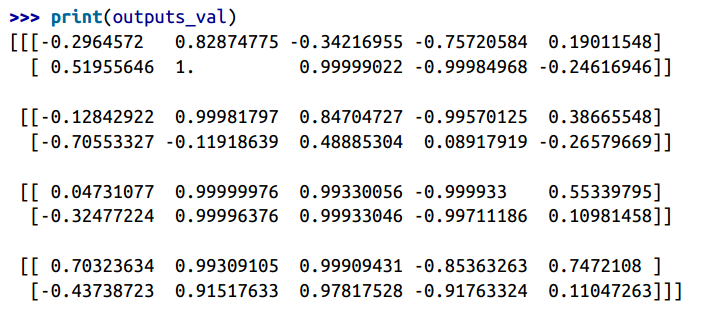## 时间上的动态展开

dynamic_rnn()函数使用while_loop()操作，在单元上运行适当的次数，如果要在反向传播期间将 GPU内 存交换到 CPU 内存，可以设置swap_memory = True，以避免内存不足错误。 方便的是，它还可以在每个时间步（形状为[None, n_steps, n_inputs]）接受所有输入的单个张量，并且在每个时间步（形状[None, n_steps, n_neurons]）上输出所有输出的单个张量。 没有必要堆叠，拆散或转置。 以下代码使用dynamic_rnn()函数创建与之前相同的 RNN。 这太好了！

import numpy as np
import tensorflow as tf
import pandas as pd

if __name__ == '__main__':
n_steps = 2
n_inputs = 3
n_neurons = 5

X = tf.placeholder(tf.float32, [None, n_steps, n_inputs])

basic_cell = tf.contrib.rnn.BasicRNNCell(num_units=n_neurons)
outputs, states = tf.nn.dynamic_rnn(basic_cell, X, dtype=tf.float32)

init = tf.global_variables_initializer()

X_batch = np.array([
[[0, 1, 2], [9, 8, 7]],  # instance 1
[[3, 4, 5], [0, 0, 0]],  # instance 2
[[6, 7, 8], [6, 5, 4]],  # instance 3
[[9, 0, 1], [3, 2, 1]],  # instance 4
])

with tf.Session() as sess:
init.run()
outputs_val = outputs.eval(feed_dict={X: X_batch})

print(outputs_val)

## 处理变长输入序列

n_steps = 2
n_inputs = 3
n_neurons = 5

reset_graph()

X = tf.placeholder(tf.float32, [None, n_steps, n_inputs])
basic_cell = tf.contrib.rnn.BasicRNNCell(num_units=n_neurons)
seq_length = tf.placeholder(tf.int32, [None])
outputs, states = tf.nn.dynamic_rnn(basic_cell, X, dtype=tf.float32,
sequence_length=seq_length)

X_batch = np.array([
# step 0     step 1
[[0, 1, 2], [9, 8, 7]], # instance 1
[[3, 4, 5], [0, 0, 0]], # instance 2 (padded with zero vectors)
[[6, 7, 8], [6, 5, 4]], # instance 3
[[9, 0, 1], [3, 2, 1]], # instance 4
])
seq_length_batch = np.array([2, 1, 2, 2])

with tf.Session() as sess:
init.run()
outputs_val, states_val = sess.run(
[outputs, states], feed_dict={X: X_batch, seq_length: seq_length_batch})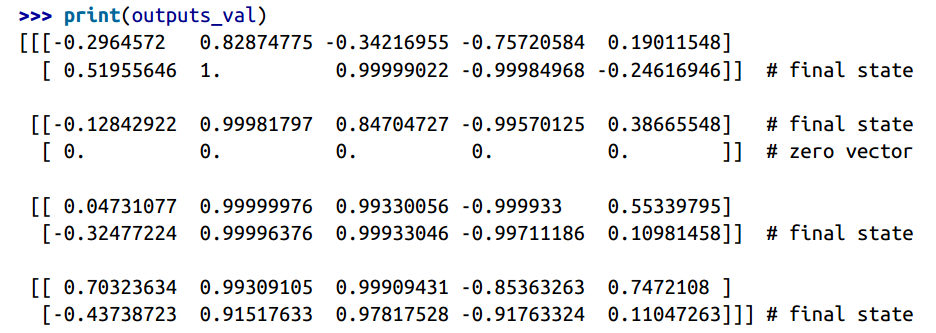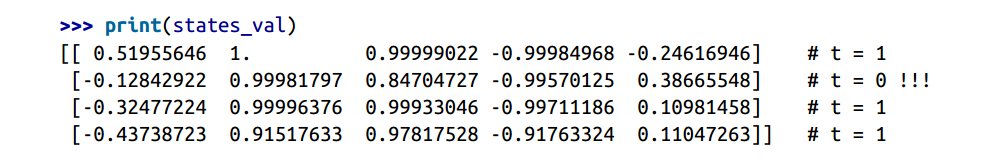## 训练 RNN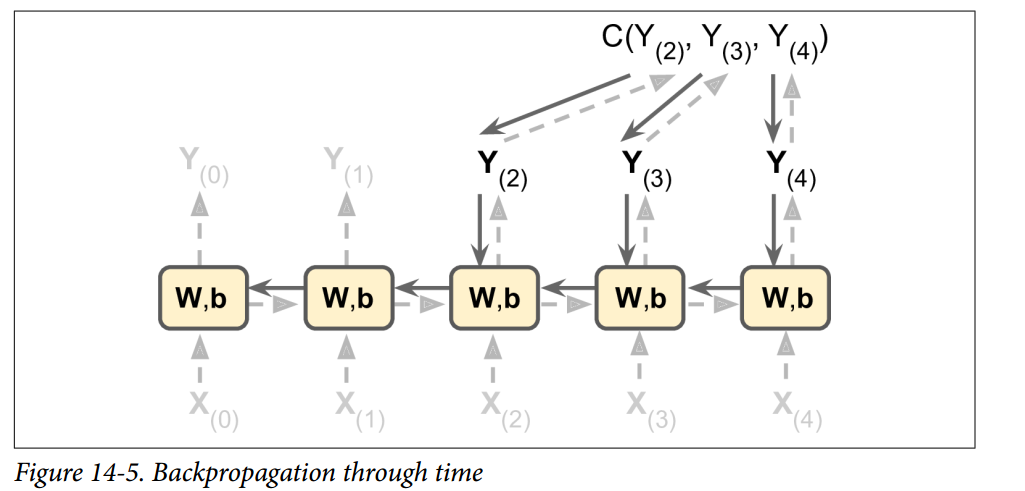## 训练序列分类器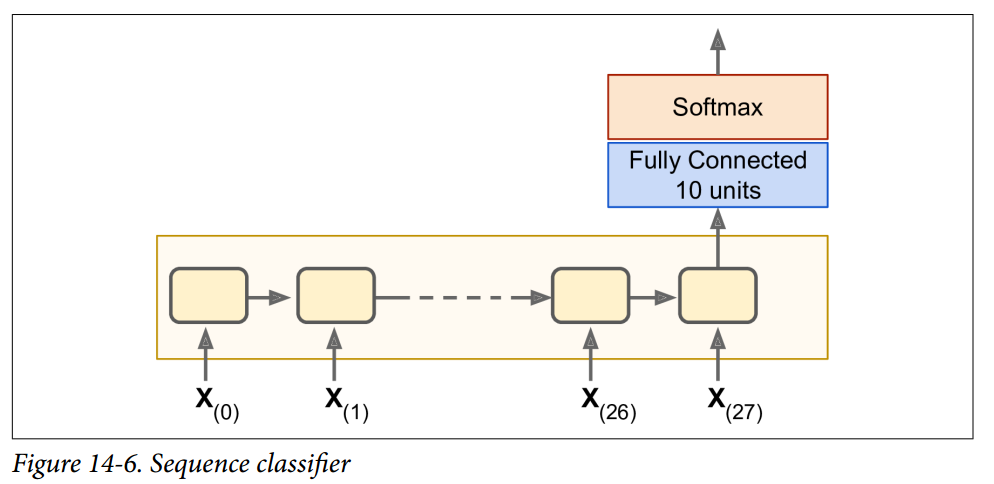n_steps = 28
n_inputs = 28
n_neurons = 150
n_outputs = 10

learning_rate = 0.001

X = tf.placeholder(tf.float32, [None, n_steps, n_inputs])
y = tf.placeholder(tf.int32, [None])

basic_cell = tf.contrib.rnn.BasicRNNCell(num_units=n_neurons)
outputs, states = tf.nn.dynamic_rnn(basic_cell, X, dtype=tf.float32)

logits = tf.layers.dense(states, n_outputs)
xentropy = tf.nn.sparse_softmax_cross_entropy_with_logits(labels=y,
logits=logits)
loss = tf.reduce_mean(xentropy)
training_op = optimizer.minimize(loss)
correct = tf.nn.in_top_k(logits, y, 1)
accuracy = tf.reduce_mean(tf.cast(correct, tf.float32))

init = tf.global_variables_initializer()

from tensorflow.examples.tutorials.mnist import input_data
X_test = mnist.test.images.reshape((-1, n_steps, n_inputs))
y_test = mnist.test.labels

batch_size = 150

with tf.Session() as sess:
init.run()
for epoch in range(n_epochs):
for iteration in range(mnist.train.num_examples // batch_size):
X_batch, y_batch = mnist.train.next_batch(batch_size)
X_batch = X_batch.reshape((-1, n_steps, n_inputs))
sess.run(training_op, feed_dict={X: X_batch, y: y_batch})
acc_train = accuracy.eval(feed_dict={X: X_batch, y: y_batch})
acc_test = accuracy.eval(feed_dict={X: X_test, y: y_test})
print(epoch, "Train accuracy:", acc_train, "Test accuracy:", acc_test)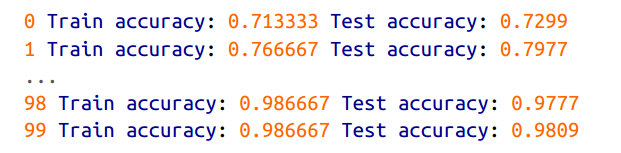## 为预测时间序列而训练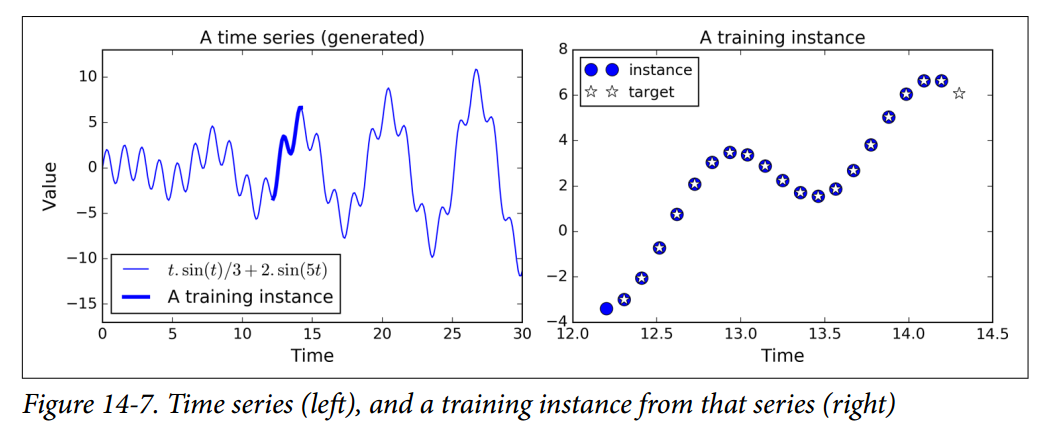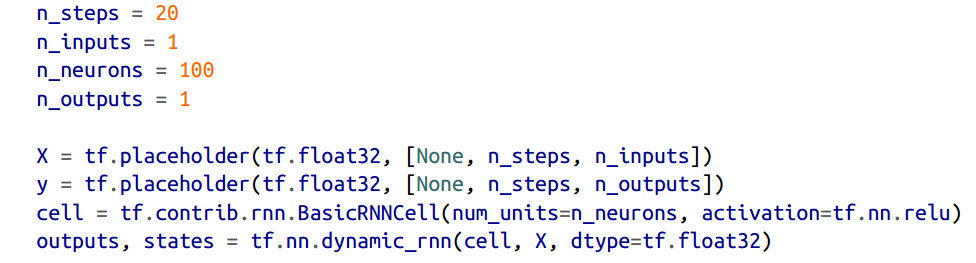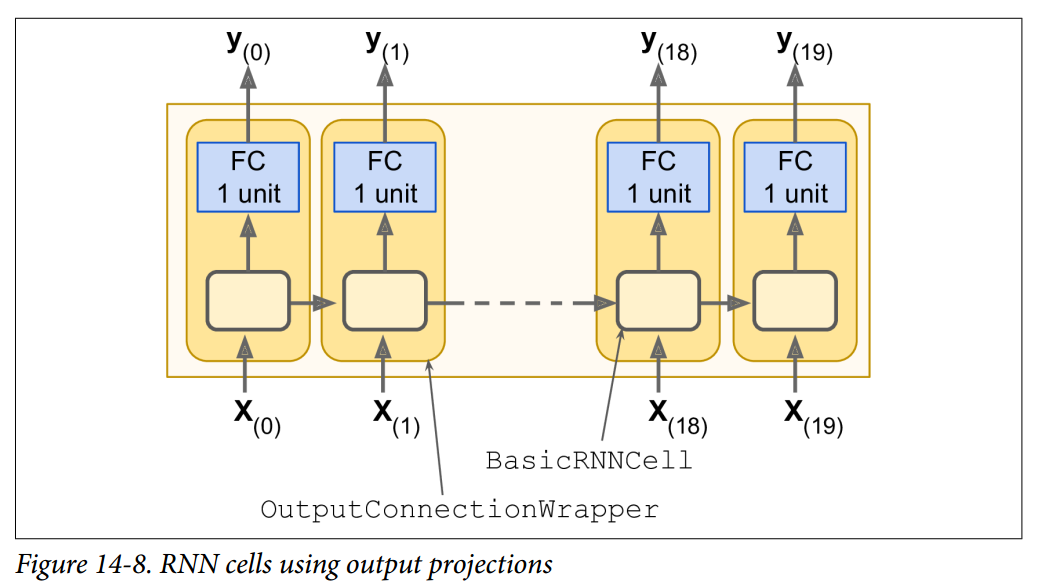cell =tf.contrib.rnn.OutputProjectionWrapper(
tf.contrib.rnn.BasicRNNCell(num_units=n_neurons,activation=tf.nn.relu),
output_size=n_outputs)

### 生成 RNNsequence = [0.] * n_steps
for iteration in range(300):
X_batch = np.array(sequence[-n_steps:].reshape(1, n_steps, 1)
y_pred = sess.run(outputs, feed_dict={X: X_batch}
sequence.append(y_pred[0, -1, 0]

## 深度 RNNn_neurons = 100
n_layers = 3

basic_cell = tf.contrib.rnn.BasicRNNCell(num_units=n_neurons)
multi_layer_cell = tf.contrib.rnn.MultiRNNCell([basic_cell] * n_layers)
outputs, states = tf.nn.dynamic_rnn(multi_layer_cell, X, dtype=tf.float32)

## Dropout 的应用

keep_prob = 0.5

cell = tf.contrib.rnn.BasicRNNCell(num_units=n_neurons)
cell_drop = tf.contrib.rnn.DropoutWrapper(cell, input_keep_prob=keep_prob)
multi_layer_cell = tf.contrib.rnn.MultiRNNCell([cell_drop]*n_layers)
rnn_outputs, states = tf.nn.dynamic_rnn(multi_layer_cell, X, dtype=tf.float32)

import sys
is_training  = (sys.argv[-1] == "train")

X = tf.placeholder(tf.float32, [None, n_steps, n_inputs])
y = tf.placeholder(tf.float32, [None, n_steps, n_outputs])
cell = tf.contrib.rnn.BasicRNNCell(num_units=n_neurons)
if is_training:
cell = tf.contrib.rnn.DropoutWrapper(cell, input_keep_prob=keep_prob)
multi_layer_cell = tf.contrib.rnn.MultiRNNCell([cell]*n_layers)
rnn_outpus, status = tf.nn.dynamic_rnn(multi_layer_cell, X, dtype=tf.float32)

[...] # bulid the rest of the graph
init = tf.global_variables_initializer()
saver = tf.train.Saver()

with tf.Session() as sess:
if is_training:
init.run()
for iteration in range(n_iterations):
[...] # train the model
save_path = saver.save(sess, "/tmp/my_model.ckpt")
else:
saver.restore(sess, "/tmp/my_model.ckpt")
[...] # use the model

## LSTM 单元

: “Long Short-Term Memory,” S.Hochreiter and J.Schmidhuber(1997)

: “Long Short-Term Memory Recurrent Neural Network Architectures for Large Scale Acoustic Modeling,” H.Sak et al.(2014)

: “Recurrent Neural Network Regularization,” W.Zaremba et al.(2015)

lstm_cell = tf.contrib.rnn.BasicLSTMCell(num_units=n_neurons)

LSTM 单元的工作机制是什么呢？在图 14-13 中展示了基本 LSTM 单元的结构。• 其中主要的全连接层输出 g(t)g(t)，它的常规任务就是解析当前的输入 x(t)x(t) 和前一时刻的短时状态 h(t1)h(t−1)。在基本形式的 RNN 单元中，就与这种形式一样，直接输出了 h(t)h(t)y(t)y(t)。与之不同的是 LSTM 单元会将一部分 g(t)g(t) 存储在长时状态中。
• 其它三个全连接层被称为门控制器（gate controller）。其采用 Logistic 作为激活函数，输出范围在 0 到 1 之间。正如在结构图中所示，这三个层的输出提供给了逐元素乘法操作，当输入为 0 时门关闭，输出为 1 时门打开。分别为：
• 遗忘门（forget gat）由 f(t)f(t) 控制，来决定哪些长期记忆需要被擦除；
• 输入门（input gate） 由 i(t)i(t) 控制，它的作用是处理哪部分 g(t)g(t) 应该被添加到长时状态中，也就是为什么被称为部分存储
• 输出门（output gate）由 o(t)o(t) 控制，在这一时刻的输出 h(t)h(t)y(t)y(t) 就是由输出门控制的，从长时状态中读取的记忆。• WxiWxiWxfWxfWxoWxoWxgWxg 是四个全连接层关于输入向量 x(t)x(t) 的权重。
• WhiWhiWhfWhfWhoWhoWhgWhg 是四个全连接层关于上一时刻的短时状态 h(t1)h(t−1) 的权重。
• bibibfbfbobobgbg 是全连接层的四个偏置项，需要注意的是 TensorFlow 将其初始化为全 1 向量，而非全 0，为了阻止网络初始训练状态下，各个门关闭从而忘记所有记忆。

### 窥孔连接

TensorFLow 中由LSTMCell实现以上变体 LSTM，并设置use_peepholes=True

lstm_cell = tf.contrib.rnn.LSTMCell(num_units=n_neurons, use_peepholes=True)

## GRU 单元• 长时状态和短时状态合并为一个向量 h(t)h(t)
• 用同一个门控制遗忘门和输入门。如果门控制输入 1，输入门打开，遗忘门关闭，反之亦然。也就是说，如果当有新的记忆需要存储，那么就必须实现在其对应位置事先擦除该处记忆。这也构成了 LSTM 本身的常见变体。
• GRU 单元取消了输出门，单元的全部状态就是该时刻的单元输出。与此同时，增加了一个控制门 r(t)r(t) 来控制哪部分前一时间步的状态在该时刻的单元内呈现。gru_cell = tf.contrib.rnn.GRUCell(n_units=n_neurons)

LSTM 或 GRU 单元是近年来 RNN 成功背后的主要原因之一，特别是在自然语言处理（NLP）中的应用。

## 自然语言处理

### 单词嵌入

vocabulary_size = 50000
embedding_size = 150
embeddings = tf.Variable(
tf.random_uniform([vocabulary_size, embedding_size], -1.0, 1.0))

train_inputs = tf.placeholder(tf.int32, shape=[None])  # from ids...
embed = tf.nn.embedding_lookup(embeddings, train_inputs)  # ...to embeddings

### 用于机器翻译的编解码器网络• 首先，到目前为止，我们已经假定所有输入序列（编码器和解码器的）具有恒定的长度。但显然句子长度可能会有所不同。有几种方法可以处理它 - 例如，使用static_rnn()dynamic_rnn()函数的sequence_length参数，来指定每个句子的长度（如前所述）。然而，教程中使用了另一种方法（大概是出于性能原因）：句子分到长度相似的桶中（例如，句子的单词 1 到 6 分到一个桶，单词 7 到 12 分到另一个桶，等等），并且使用特殊的填充标记（例如"<pad>"）来填充较短的句子。例如，"I drink milk"变成"<pad> <pad> <pad> milk drink I"，翻译成"Je bois du lait <eos> <pad>"。当然，我们希望忽略任何 EOS 标记之后的输出。为此，本教程的实现使用target_weights向量。例如，对于目标句子"Je bois du lait <eos> <pad>"，权重将设置为[1.0,1.0,1.0,1.0,1.0,0.0]（注意权重 0.0 对应目标句子中的填充标记）。简单地将损失乘以目标权重，将消除对应 EOS 标记之后的单词的损失。

• 其次，当输出词汇表很大时（就是这里的情况），输出每个可能的单词的概率将会非常慢。 如果目标词汇表包含 50,000 个法语单词，则解码器将输出 50,000 维向量，然后在这样的大向量上计算 softmax 函数，计算量将非常大。 为了避免这种情况，一种解决方案是让解码器输出更小的向量，例如 1,000 维向量，然后使用采样技术来估计损失，而不必对目标词汇表中的每个单词计算它。 这种采样 Softmax 技术是由 SébastienJean 等人在 2015 年提出的。在 TensorFlow 中，你可以使用sampled_softmax_loss()函数。

• 第三，教程的实现使用了一种注意力机制，让解码器能够窥视输入序列。 注意力增强的 RNN 不在本书的讨论范围之内，但如果你有兴趣，可以关注机器翻译，机器阅读和图像说明的相关论文。

• 最后，本教程的实现使用了tf.nn.legacy_seq2seq模块，该模块提供了轻松构建各种编解码器模型的工具。 例如，embedding_rnn_seq2seq()函数会创建一个简单的编解码器模型，它会自动为你处理单词嵌入，就像图 14-15 中所示的一样。 此代码可能会很快更新，来使用新的tf.nn.seq2seq模块。

## 练习

1. 你能想象 seq2seq RNN 的几个应用吗？ seq2vec 的 RNN 呢？vex2seq 的 RNN 呢？
2. 为什么人们使用编解码器 RNN 而不是简单的 seq2seq RNN 来自动翻译？
3. 如何将卷积神经网络与 RNN 结合，来对视频进行分类？
4. 使用dynamic_rnn()而不是static_rnn()构建 RNN 有什么好处？
5. 你如何处理长度可变的输入序列？ 那么长度可变输出序列呢？
6. 在多个 GPU 上分配深层 RNN 的训练和执行的常见方式是什么？
7. Hochreiter 和 Schmidhuber 在其关于 LSTM 的文章中使用了嵌入式 Reber 语法。 它们是产生字符串，如"BPBTSXXVPSEPE"的人造语法。查看 Jenny Orr 对此主题的不错的介绍。 选择一个特定的嵌入式 Reber 语法（例如 Jenny Orr 页面上显示的语法），然后训练一个 RNN 来确定字符串是否遵循该语法。 你首先需要编写一个函数，该函数能够生成训练批量，包含大约 50% 遵循语法的字符串，以及 50% 不遵循的字符串。
8. 解决“How much did it rain? II”（下雨下了多久 II）Kaggle 比赛。 这是一个时间序列预测任务：它为你提供极化雷达值的快照，并要求预测每小时降水量。 Luis Andre Dutra e Silva 的采访对他在比赛中获得第二名的技术，提供了一些有趣的见解。 特别是，他使用了由两个 LSTM 层组成的 RNN。
9. 通过 TensorFlow 的 Word2Vec 教程来创建单词嵌入，然后通过 Seq2Seq 教程来训练英法翻译系统。

apachecn_飞龙
+ 关注